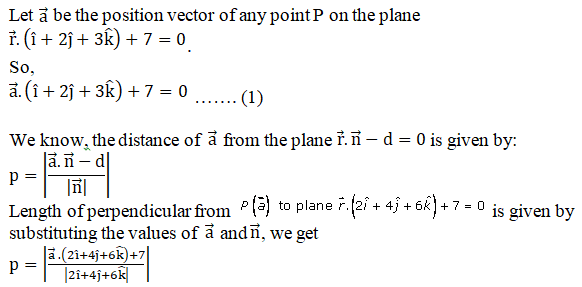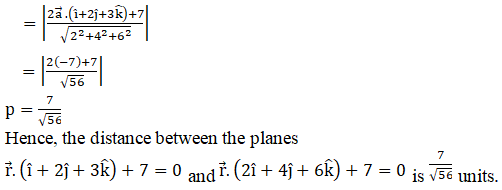# RD Sharma Solutions Class 12 The Plane Exercise 29.10

RD Sharma Solutions for Class 12 Maths Chapter 29 The Plane Exercise 29.10, is given here for students to prepare and score well in the board exams. Students can refer to these solutions and can follow the correct methodology for solving problems and can also clear their doubts pertaining to any question. Students are advised to practice the RD Sharma Class 12 Solutions on a regular basis which yields good results in their exams. Download the solutions pdf for free from their respective links.

## Download PDF of RD Sharma Solutions For Class 12 Maths Chapter 29 Exercise 10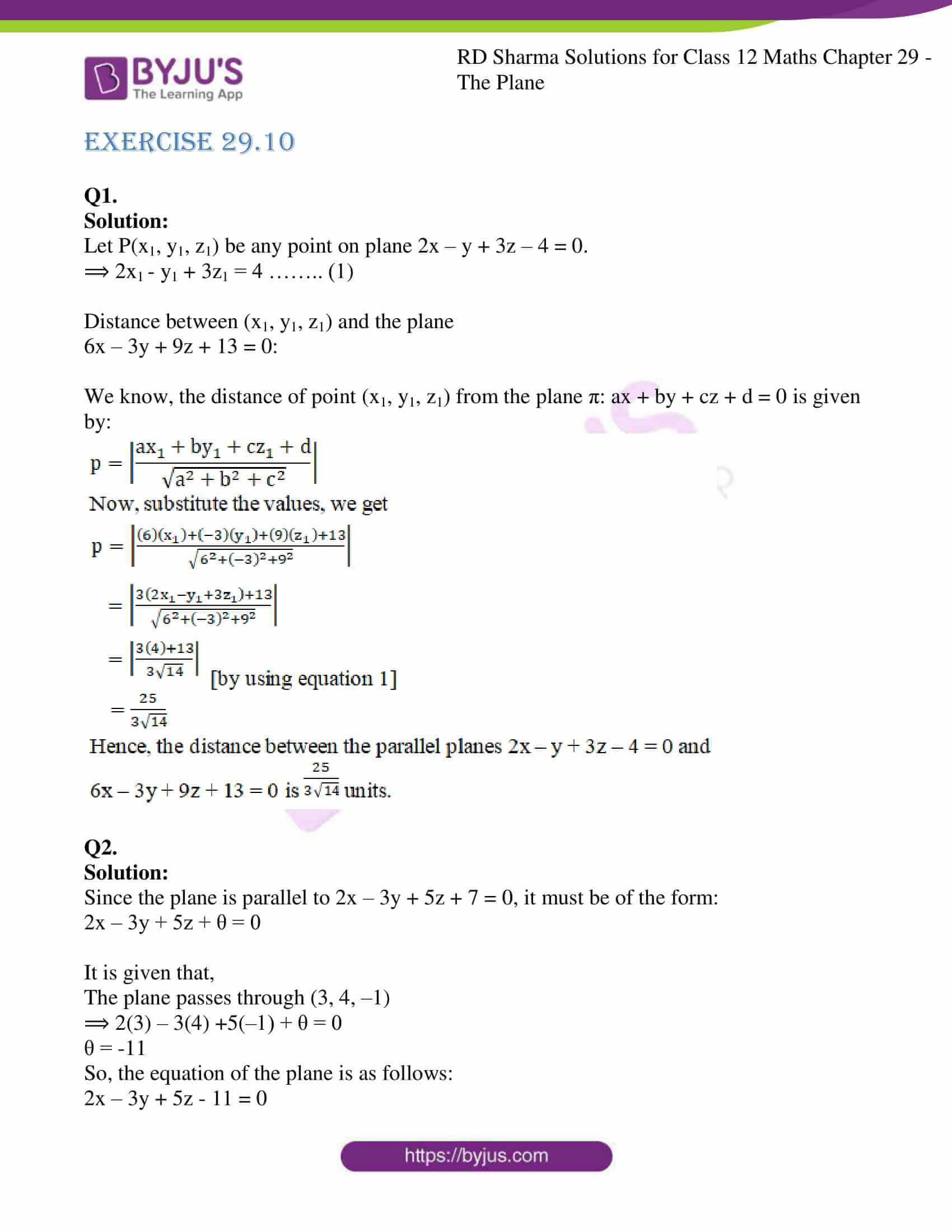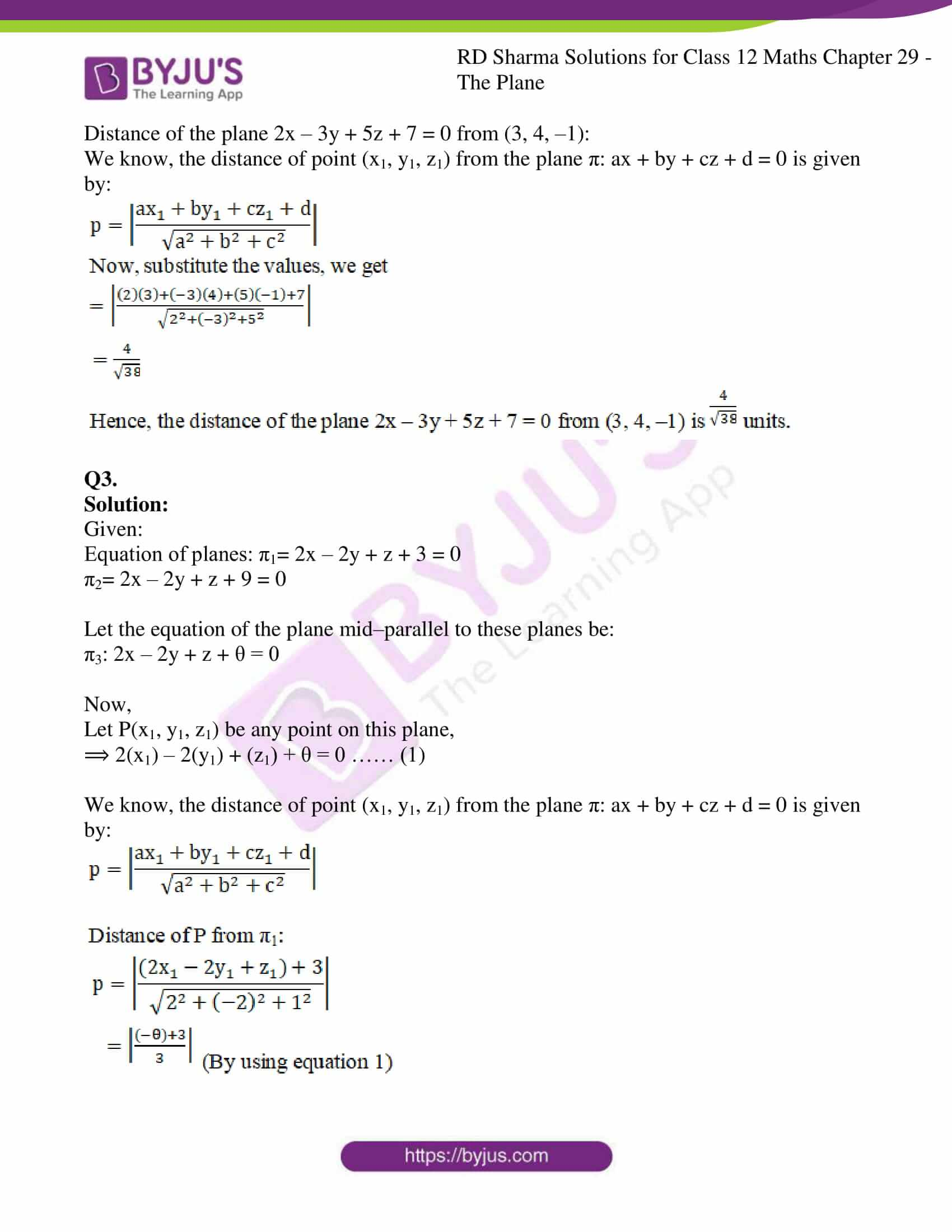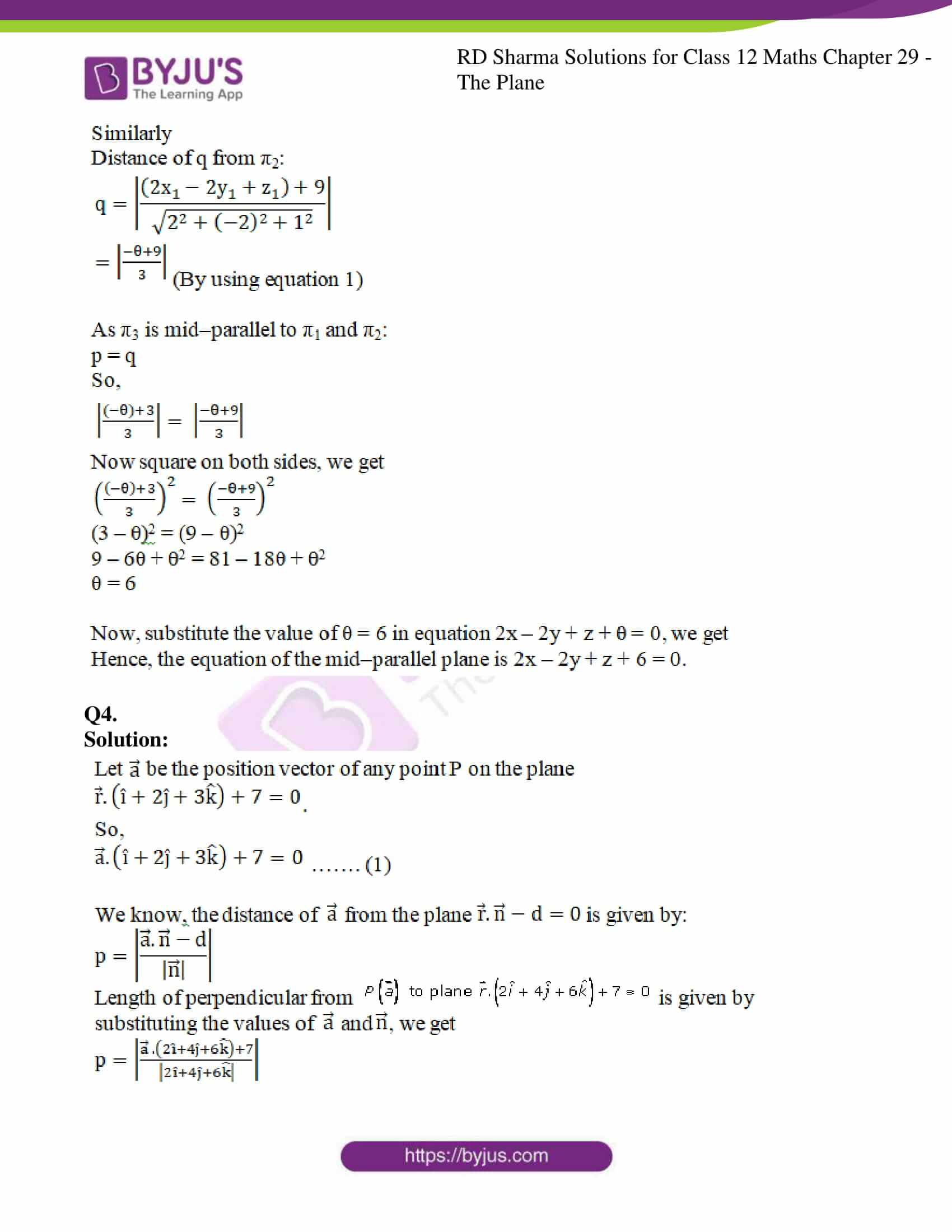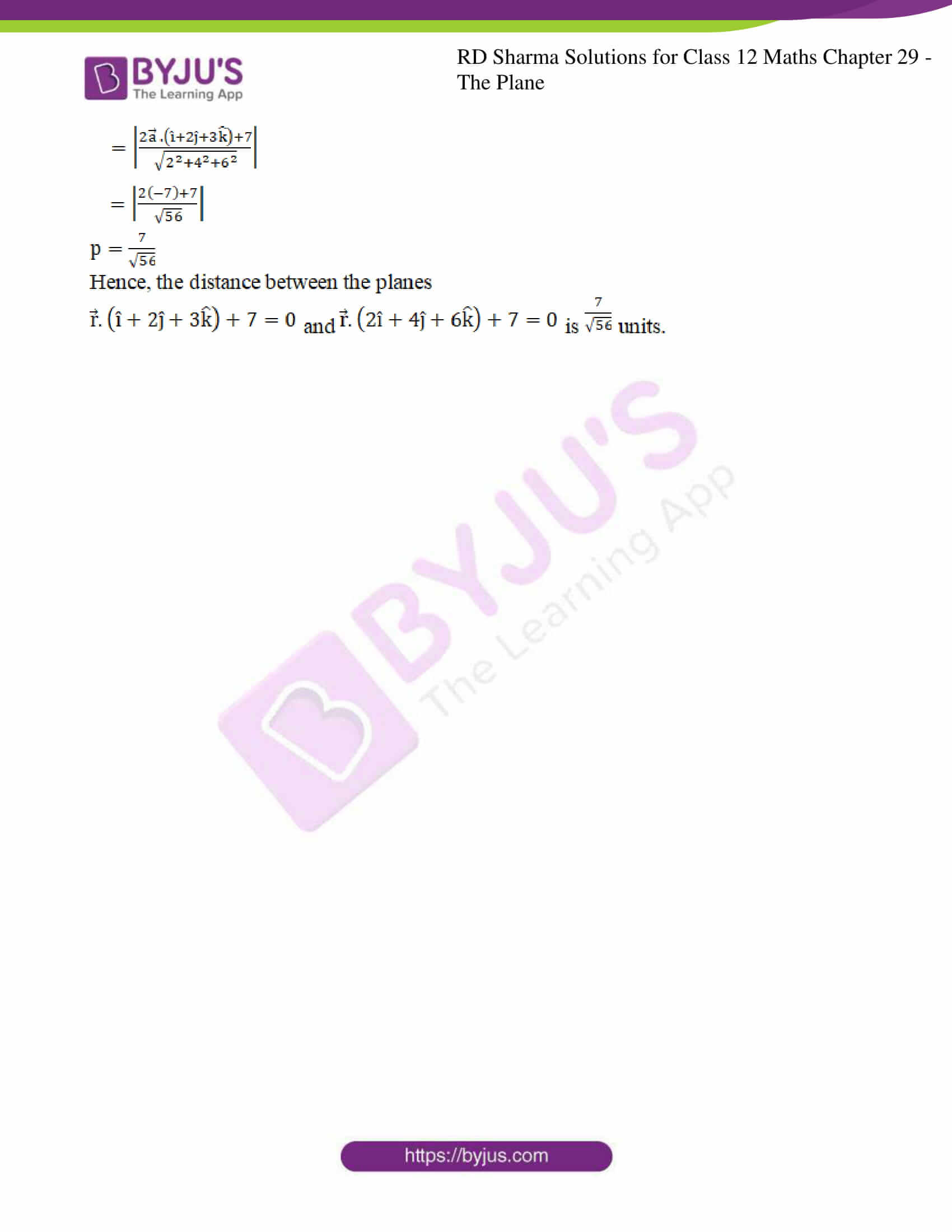### Access RD Sharma Solutions For Class 12 Maths Chapter 29 Exercise 10

EXERCISE 29.10

Q1.

Solution:

Let P(x1, y1, z1) be any point on plane 2x – y + 3z – 4 = 0.

⟹ 2x1 – y1 + 3z1 = 4 …….. (1)

Distance between (x1, y1, z1) and the plane

6x – 3y + 9z + 13 = 0:

We know, the distance of point (x1, y1, z1) from the plane π: ax + by + cz + d = 0 is given by: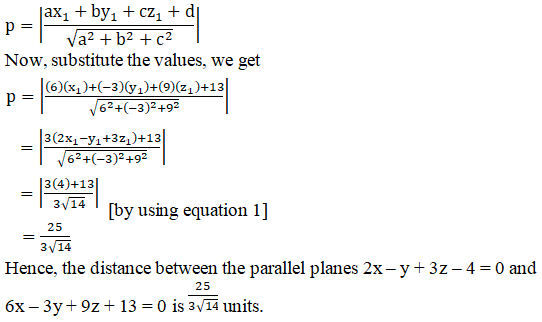Q2.

Solution:

Since the plane is parallel to 2x – 3y + 5z + 7 = 0, it must be of the form:

2x – 3y + 5z + θ = 0

It is given that,

The plane passes through (3, 4, –1)

⟹ 2(3) – 3(4) +5(–1) + θ = 0

θ = -11

So, the equation of the plane is as follows:

2x – 3y + 5z – 11 = 0

Distance of the plane 2x – 3y + 5z + 7 = 0 from (3, 4, –1):

We know, the distance of point (x1, y1, z1) from the plane π: ax + by + cz + d = 0 is given by: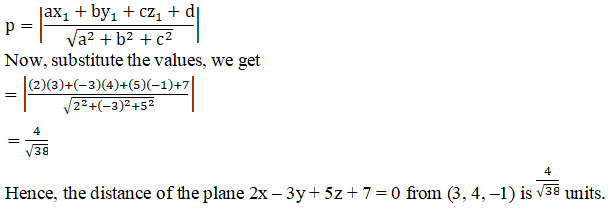Q3.

Solution:

Given:

Equation of planes: π1= 2x – 2y + z + 3 = 0

π2= 2x – 2y + z + 9 = 0

Let the equation of the plane mid–parallel to these planes be:

π3: 2x – 2y + z + θ = 0

Now,

Let P(x1, y1, z1) be any point on this plane,

⟹ 2(x1) – 2(y1) + (z1) + θ = 0 …… (1)

We know, the distance of point (x1, y1, z1) from the plane π: ax + by + cz + d = 0 is given by: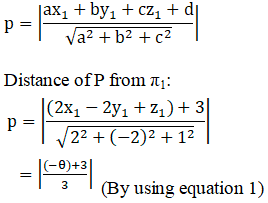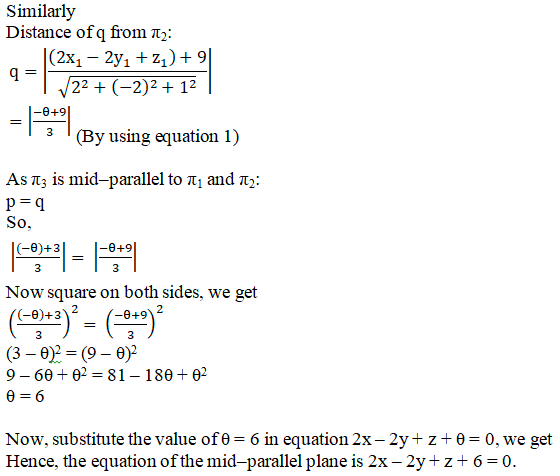Q4.

Solution: